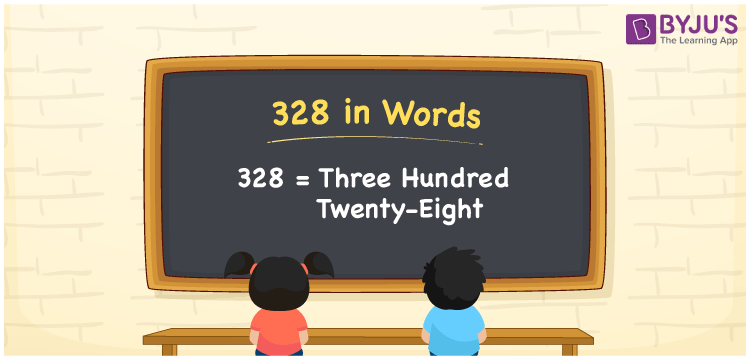# 328 in Words

328 in words is written as Three hundred twenty-eight. In both the International System of Numerals and the Indian System of Numerals, 328 is written as Three hundred twenty-eight. The number 328 is a Cardinal Number as it represents some quantity. For example, “328 students have participated in the test”.

 328 in Words Three hundred twenty-eight Three hundred twenty-eight in Number 328

## 328 in English Words

We write 328 in English Words using the letters of the English alphabet. Therefore, we read 328 in English as “Three hundred twenty-eight.”## How to Write 328 in Words?

To write 328 in words, we shall use the place value chart. In the place value chart, write 3 in the hundreds, 2 in the tens, and 8 in the ones, respectively. Now let us make a place value chart to write the number 328 in words.

 Hundreds Tens Ones 3 2 8

Thus, we can write the expanded form as

3 × Hundred + 2 × Ten + 8 × One

= 3 × 100 + 2 × 10 + 8 × 1

= 300 + 20 + 8

= 328

= Three hundred twenty-eight.

328 is a natural number, the successor of 327 and the predecessor of 329.

328 in words – Three hundred twenty-eight

• Is 328 an odd number? – No
• Is 328 an even number? – Yes
• Is 328 a perfect square number? – No
• Is 328 a perfect cube number? – No
• Is 328 a prime number? – No
• Is 328 a composite number? – Yes

## Frequently Asked Questions on 328 in Words

Q1

### How to write 328 in words?

328 in words is written as Three hundred twenty-eight.
Q2

### How to write 328 in the International and Indian System of Numerals?

In both, the system of numerals, 328 in words, is written as Three hundred twenty-eight.
Q3

### What is the preceding number of 328?

The number that precedes 328 is 327.